# NCERT SOLUTION FOR CLASS 8 MATHS CHAPTER 13 DIRECT AND INVERSE PROPORTIONS

The National Council of Educational Research and Training (NCERT) is an autonomous body of the Indian government that formulates the curricula for schools in India that are governed by the Central Board of Secondary Education (CBSE) and certain state boards. Therefore, students who will be taking the Class 10 tests administered by various boards should consult this NCERT Syllabus in order to prepare for those examinations, which in turn will assist those students get a passing score.

You can also download the NCERT SOLUTION FOR CLASS 8 MATHS CHAPTER 13 DIRECT AND INVERSE PROPORTIONS to assist you in comprehensively reviewing the course material and achieving a higher score on your upcoming assessments.

Students are able to create a strong conceptual basis with the help of the NCERT Solutions PDFs available at SWC. This base plays a key part in the students’ preparation for the later phases of the competitive examinations. We give comprehensive answers to challenges posed by NCERT in accordance with the methodology provided by CBSE. The students are able to effortlessly prepare all of the ideas that are presented in their respective courses better and more successfully, and they are even able to succeed in passing the most difficult competitive examinations, such as JEE Main, JEE Advanced, NEET, AIMS, and so on.

Your academic journey begins a new stage as you reach the eighth grade, and a new facet of learning awaits you. This is the year where pupils begin to acquire an interest in the topic at hand, and it is also the year in which you choose whether you will study business, humanity, or science in the following year (class 12). As a consequence of this, having a solid foundation of knowledge in fields such as mathematics and physics comes highly recommended. The NCERT textbook for class 8 is the greatest book now available on the market for enhancing one’s education in the areas of mathematics and science. Read up on the theory that is presented in the book for grade 8 and answer all of the questions that are posed in the practice section of the NCERT textbook.

## NCERT SOLUTION FOR CLASS 8 MATHS CHAPTER 13 DIRECT AND INVERSE PROPORTIONS – Exercises

### NCERT Solutions for Class 8 Maths Exercise 13.1

Question 1.

Following are he car parking charges near a railway station up to:

4 hours   Rs.60

8 hours   Rs.100

12 hours   Rs.140

24 hours   Rs.180

Check if the parking charges are in direct proportion to the parking time.

Solution :

Charges per hour:

Therefore, the parking charges are not in direct proportion to the parking time.

Question 2.

A mixture of paint is prepared by mixing 1 part of red pigments with 8 parts of base. In the following table, find the parts of base that need to be added.

Solution :

Question 3.

In Question 2 above, if 1 part of a red pigment requires 75 mL of base, how much red pigment should we mix with 1800 mL of base?

Solution :

Let the parts of red pigment mix with 1800 mL base be x.

Since it is in direct proportion.

Hence with base 1800 mL, 24 parts red pigment should be mixed.

Question 4.

A machine in a soft drink factory fills 840 bottles in six hours. How many bottles will it fill in five hours?

Solution :

Let the number of bottles filled in five hours be x.

Here ratio of hours and bottles are in direct proportion.

Hence machine will fill 700 bottles in five hours.

Question 5.

A photograph of a bacteria enlarged 50,000 times attains a length of 5 cm as shown in the diagram. What is the actual length of the bacteria? If the photograph is enlarged 20,000 times only, what would be its enlarged length?

Solution :

Let enlarged length of bacteria be x.

Actual length of bacteria

=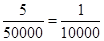cm =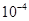cm

Here length and enlarged length of bacteria are in direct proportion.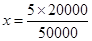⇒= 2 cm

Hence the enlarged length of bacteria

is 2 cm.

Question 6.

In a model of a ship, the mast is 9 cm high, while the mast of the actual ship is 12 m high. If the length if the ship is 28 m, how long is the model ship?

Solution :

Let the length of model ship be x.

Here length of mast and actual length of ship are in direct proportion.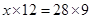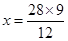= 21 cm

Hence length of the model ship is 21 cm.

Question 7.

Suppose 2 kg of sugar contains 9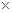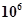crystals. How many sugar crystals are there in (i) 5 kg of sugar? (ii) 1.2 kg of sugar?

Solution :

(i) Let sugar crystals be x.

Hence the number of sugar crystals is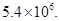Question 8.

Rashmi has a road map with a scale of 1 cm representing 18 km. She drives on a road for 72 km. What would be her distance covered in the map?

Solution :

Let distance covered in the map be x.

Here actual distance and distance covered in the map are in direct proportion.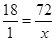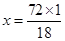= 4 cm

Hence distance covered in the map is 4 cm.

Question 9 .

A 5 m 60 cm high vertical pole casts a shadow 3 m 20 cm long. Find at the same time (i) the length of the shadow cast by another pole 10 m 50 cm high (ii) the height of a pole which casts a shadow 5 m long.

Solution :

Here height of the pole and length of the shadow are in direct proportion.

And 1 m = 100 cm

5 m 60 cm = 5100 + 60 = 560 cm

3 m 20 cm = 3100 + 20 = 320 cm

10 m 50 cm = 10100 + 50 = 1050 cm

5 m = 5100 = 500 cm

(i) Let the length of the shadow of another pole be x.

= 875 cm = 8 m 75 cm

Hence height of the pole is 8 m 75 cm.

Question 10.

A loaded truck travels 14 km in 25 minutes. If the speed remains the same, how far can it travel in 5 hours?

Solution :

Let distance covered in 5 hours be x km.

∵ 1 hour = 60 minutes

∴ 5 hours = 560 = 300 minutes

Here distance covered and time in direct proportion.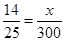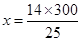= 168 km

### NCERT Solutions for Class 8 Maths Exercise 13.2

Question 1.

Which of the following are in inverse proportion:

(i) The number of workers on a job and the time to complete the job.

(ii) The time taken for a journey and the distance travelled in a uniform speed.

(iii) Area of cultivated land and the crop harvested.

(iv) The time taken for a fixed journey and the speed of the vehicle.

(v) The population of a country and the area of land per person.

Solution :

(i) The number of workers and the time to complete the job is in inverse proportion

because less workers will take more time to complete a work and more workers will take less time to complete the same work.

(ii) Time and distance covered in direct proportion.

(iii) It is a direct proportion because more are of cultivated land will yield more crops.

(iv) Time and speed are inverse proportion because if time is less, speed is more.

(v) It is a inverse proportion. If the population of a country increases, the area of land per person decreases.

Question 2.

In a Television game show, the prize money of Rs.1,00,000 is to be divided equally amongst the winners. Complete the following table and find whether the prize money given to an individual winner is directly or inversely proportional to the number of winners:

Solution :

Here number of winners and prize money are in inverse proportion because winners are increasing, prize money is decreasing.

When the number of winners are 4, each winner will get == Rs. 25,000

When the number of winners are 5, each winner will get = 100000/5= Rs. 20,000

When the number of winners are 8, each winner will get =100000/8 = Rs. 12,500

When the number of winners are 10, each winner will get = 100000/10 = Rs. 10,000

When the number of winners are 20, each winner will get = 100000/20 = Rs.  5,000

Question 3.

Rehman is making a wheel using spokes. He wants to fix equal spokes in such a way that the angles between any pair of consecutive spokes are equal. Help him by completing the following table:

(i) Are the number of spokes and the angles formed between the pairs of consecutive spokes in inverse proportion?

(ii) Calculate the angle between a pair of consecutive spokes on a wheel with 15 spokes.

(iii) How many spokes would be needed, if the angle between a pair of consecutive spokes is 40°?

Solution :

Here the number of spokes are increasing and the angle between a pair of consecutive spokes is decreasing. So, it is a inverse proportion and angle at the centre of a circle is  360°.

When the number of spokes is 8, then angle between a pair of consecutive spokes = 360°/8 = 45°

When the number of spokes is 10, then angle between a pair of consecutive spokes= 360°/10 = 36°

When the number of spokes is 12, then angle between a pair of consecutive spokes= 360°/12 = 30°

(i) Yes, the number of spokes and the angles formed between a pair of consecutive spokes is in inverse proportion.

(ii) When the number of spokes is 15, then angle between a pair of consecutive spokes= .360°/15 = 24°

(iii) The number of spokes would be needed = 360°/40 = 9°

Question 4.

If a box of sweets is divided among 24 children, they will get 5 sweets each. How many would each get, if the number of the children is reduced by 4?

Solution :

∵ Each child gets = 5 sweets

∴ 24 children will get 24 × 5 = 120 sweets

Total number of sweets = 120

If the number of children is reduced by 4, then children left = 24 – 4 = 20

Now each child will get sweets = 120 / 20

= 6 sweets

Question 5.

A farmer has enough food to feed 20 animals in his cattle for 6 days. How long would the food last if there were 10 more animals in his cattle?

Solution :

Let the number of days be x.

Total number of animals = 20 + 10 = 30

Here the number of animals and the number of days are in inverse proportion.

Hence the food will last for four days.

Question 6.

A contractor estimates that 3 persons could rewire Jasminder’s house in 4 days. If, he uses 4 persons instead of three, how long should they take to complete the job?

Solution :

Let time taken to complete the job be x.

Hence they will complete the job in 3 days.

Question 7.

A batch of bottles was packed in 25 boxes with 12 bottles in each box. If the same batch is packed using 20 bottles in each box, how many boxes would be filled?

Solution :

Let the number of boxes be x.

Here the number of bottles and the number of boxes are in inverse proportion.

∴  12 /20 = x /25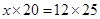⇒   x  12 × 25 / 20= 15

Hence 15 boxes would be filled.

Question 8.

A factory requires 42 machines to produce a given number of articles in 63 days. How many machines would be required to produce the same number of articles in 54 days?

Solution :

Let the number of machines required be x.

Here the number of machines and the number of days are in inverse proportion.

Hence 49machineswould be required.

Question 9.

A car takes 2 hours to reach a destination by travelling at the spe^ed of 60 km/hr. How long will it take when the car travels at the speed of 80 km/hr?

Solution :

Let the number of hours be x.

to reach its destination.

Question 10.

Two persons could fit new windows in a house in 3 days.

(i) One of the persons fell ill before the work started. How long would the job take now?

(ii) How many persons would be needed to fit the windows in one day?

Solution :

(i) Let the number of days be x.

Here the number of persons and the number of days are in inverse proportion.

⇒   x × 1 = 2 × 3

⇒   x = 2×3 /1= 6 days

(ii)  Let the number of persons be x.

Here the number of persons and the number of days are in inverse proportion.

= 6 persons

Question 11.

A school has 8 periods a day each of 45 minutes duration. How long would each period be, if the school has 9 periods a day, assuming the number of school hours to be the same?

Solution :

Let the duration of each period be x.

Here the number of periods and the duration of periods are in inverse proportion.

Hence duration of each period would be 40 minutes.

## Conclusions for NCERT Solutions for Class 8 Chapter 13

SWC academic staff has developed NCERT answers for the 13th chapter of the eighth-grade mathematics curriculum. We have solutions prepared for all of the exercises in the 13th chapter. The answers, broken down into steps, to all of the questions included in the NCERT textbook’s first chapter are provided here. Read the 13th chapter on theory. Be certain that you have read the theory section of chapter13 of the NCERT textbook and that you have learned the formulas for the chapter that you are studying.

When working through the exercises in the NCERT textbook, if you run into any type of difficulty or uncertainty, you may use the swc NCERT Solutions for class 8 as a point of reference. While you are reading the theory form textbook, it is imperative that you always have notes prepared. You should make an effort to understand things from the very beginning so that you may create a solid foundation in the topic. Use the NCERT as your parent book to ensure that you have a strong foundation. After you have finished reading the theoretical section of the textbook, you should go to additional reference books.